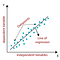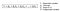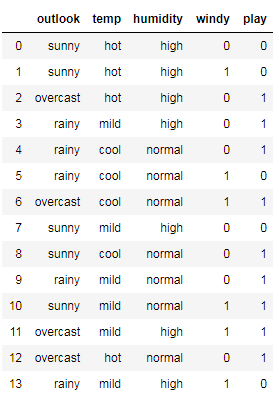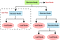# Ordinary Least Squares in Linear Regression

In Linear Regression problems, there are two main approaches for estimating parameters.

1. Mathematic Approach or Ordinary Least Squares.
2. Optimization Algorithm using Gradient Descent Algorithm.

The first method Ordinary Least Squares uses Linear Algebra operations to estimate the parameters while the Optimization method uses the Gradient descent to estimate the model’s parameters or coefficients.

In this article, I am going to talk about the Least Squares Approach to estimate the parameters for simple and multiple linear regression.

# For Simple Linear Regression

Simple linear regression is a linear regression model in which we use one feature(also called column or independent variable) to predict the target (also…

# Evaluation Metrics for Classification Problems | Machine Learning

In the last article, I have talked about Evaluation Metrics for Regression, and In this article, I am going to talk about Evaluation metrics for Classification problems.

1. Accuracy
2. Precision
3. Recall
4. F1-Score

# Classification Problems:

In Classification problems, we try to predict and to identifying which of a set of categories a new observation belongs to, For Example; assigning a given email to the “spam” or “non-spam” class.

Before getting into the evaluation metrics, we have first to know about Confusion Matrix, which is a specific table layout that allows visualization of the performance of an algorithm and also to…

# Evaluation Metrics for Regression Problems| Machine Learning

In this article, I am going to talk about some Evaluation metrics for Regression problems.

1. R-Squared
2. Mean Absolute Error
3. Mean Squared Error

# Regression Problems:

In Regression problems, we try to predict continuous values, for example; predicting House prices.Linear Regression in Machine Learning

So, as a Data Scientist, you can create a model to predict those values, you have to evaluate your model, in other words, you have to compute some metrics and based of them you can check if your model is good enough or not.

R-Squared is one of these metrics, which you can use to evaluate your model

# Download Youtube Videos using youtube_dl module in Python

You might want to store some video for future use where it will be required due to lack of internet or saving data or any other reason.

What if we tell you that you can do this exact very thing using Python. Let’s see how to download Youtube videos using youtube_dl module in Python.

youtube-dl is a command-line program to download videos from YouTube.com and a few more sites. It requires the Python interpreter, version 2.6, 2.7, or 3.2+, and it is not platform specific. It should work on your Unix box, on Windows or on macOS. …

# Creating a Simple Memory Game in Python using Tkinter

In this article, I am going to show you how to create a simple memory game using Tkinter GUI in python.

Python provides various options for developing graphical user interfaces (GUIs). The most important are listed below.

Tkinter − Tkinter is the Python interface to the Tk GUI toolkit shipped with Python. We would look at this option in this article.

wxPython − This is an open-source Python interface for wxWindows http://wxpython.org.

JPython − JPython is a Python port for Java which gives Python scripts seamless access to Java class libraries on the local machine http://www.jython.org.

N.B: There are many…

# Maximum Likelihood Estimation and Logistic Regression From Scratch

Logistic regression is a generalized linear model that we can use to model or predict categorical outcome variables. For example, we might use logistic regression to predict whether a tumor is malignant or benign, but probably not to predict the price of someone’s house.

How are we going to do so? Well, In Logistic Regression, we’re essentially trying to find the weights(coefficients) that maximize the likelihood of producing our given data and use them to categorize the response variable

Since the likelihood maximization in logistic regression doesn’t have a closed-form solution, I’ll solve the optimization problem with gradient ascent. Gradient…

# Gradient Descent Algorithm From Scratch using Python

In this article, I am going to implement the Gradient Descent Algorithm from scratch in Python,

1- What is Linear Regression?
2- Cost Function or Loss Function
3- Gradient Descent Algorithm
4- Implementing the python code for Gradient Descent Algorithm

Without further ado, let’s dive in.

Linear Regression is a Supervised Learning Algorithm that predicts continuous values, for example: Predicting house prices.
It is a linear approach to modeling the relationship between the dependent variable (Y) and one(Simple Linear Regression) or more(Multiple Linear Regression) independent variables (X).

We will define a linear relationship between these two variables as follows:Linear Regression Formula

Cost…

# Gini Gain vs Gini Impurity | Decision Tree — A Simple Explanation

Gini Impurity, like Information Gain and Entropy, is just a metric used by Decision Tree Algorithms to measure the quality of a split.

You may like to watch this article as a video? Check it out!

Let’s consider the Dataset below,Question: We would like to build a decision tree from the Dataset, how can we choose the best feature to split the data and how can we measure our splitting?

This is where our metric “ Gini Impurity ” comes in, Gini Impurity measures the randomness in our data, how random our data is?

Gini Impurity Formula:

If…

# A Simple Explanation of Entropy and Information Gain | Decision Tree Classification | Machine Learning

What Entropy and Information Gain are? and how they are used to decide which attribute should be selected as the decision node?

In a Decision Tree Classification Algorithm, the classification is done by splitting the dataset into a bunch of branches based on decision nodes that determine where to go or what the result will be.Decision Tree Classification Flowchart

Once you see the image above, you may ask: “How does a Decision Tree Algorithm decide where to split?” or “How does it decide the best attribute(Decision Node) ?”

Well, to answer that question let’s take a simple example to work on.

suppose you…## Idriss Jairi

Computer Science student and passionate